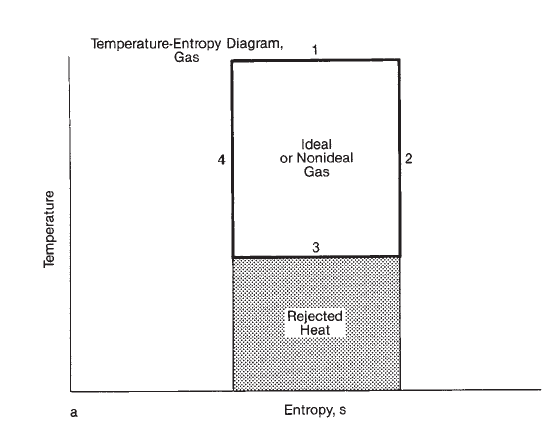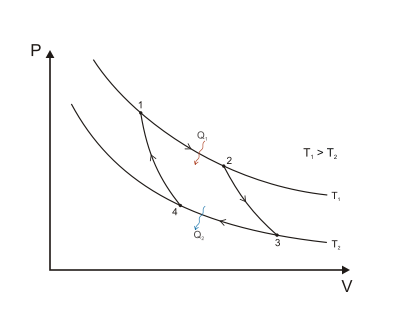Thermodynamics

# Carnot Thermodynamic Cycle

Thermodynamic cycles are a combination of processes so that heat may be converted to work on a continuous basis. Some of the most basic thermodynamic cycles are presented below

### Carnot cycle

Carnot cycle has no actual counterpart in real life. Despite the fact that it is purely theoretical thermodynamic cycle, it is mostly used for illustrating the basic principles of thermodynamics. It is represented in a temperature (T)-entropy (s) chart.

The Carnot cycle consists of the following processes:

1. Heat is added to the working medium at constant temperature (isothermal process, dT=0), resulting in expansion work.
2. Adiabatic isentropic (ds=0) expansion occurs with expansion work. A thermodynamic process in which the net heat transfer to or from the working fluid is zero is called adiabatic.
3. Heat is rejected to the surrounding environment at a constant temperature.
4. Adiabatic isentropic compression occurs back to the starting temperature with compression work.### Typical Carnot cycle for a gas

In theory, because its processes are reversible (a cycle is called reversible when it can be reversed without any loss of energy: in practice, a fully reversible process does not exist). The Carnot cycle offers the maximum thermodynamic efficiency attainable between any given temperatures of heat source and heat sink.

### Carnot cycle efficiency definition

Carnot cycle efficiency is defined as the ratio of the net work output produced to the total heat input. Referring to the T-S diagram shown below, efficiency of a cyclic process depends entirely on the temperatures at which heat addition and rejection occur, i.e:

η= (T1-T2)/T1 = 1 - T2/T1,

where T1 is the absolute temperature of the heat source (in K) and T2 is the absolute temperature of the heat sink (in K).A Carnot cycle acting as a heat engine, illustrated on a pressure-volume diagram to illustrate the work done